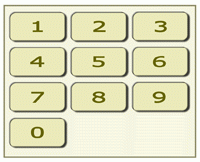### Problem Statement

This problem contains an image that can be viewed in the applet.

A door of a safe is locked by a password. Josh witnessed an employee opening the safe. Here is the information Josh spied.

1. The password is a sequence containing exactly N digits..
2. The password is entered using the keypad shown in the picture below.
3. Every pair of neighboring digits in the password is adjacent on the keypad. Two digits are adjacent on the keypad if they are distinct and have a common edge.Josh has evil intentions of unsealing the safe, but before he can realize his plan, he wants to know how many different passwords exist. Given the value for N, return the number of possible passwords that satisfy all the constraints given above.

### Definition

 Class: UnsealTheSafe Method: countPasswords Parameters: int Returns: long Method signature: long countPasswords(int N) (be sure your method is public)

### Constraints

-N will be between 2 and 30, inclusive.

### Examples

0)

 `2`
`Returns: 26`
 if the first button is 1, the second button can be either 2 or 4 if the first button is 2, the second button can be either 1, 3 or 5 if the first button is 3, the second button can be either 2 or 6 if the first button is 4, the second button can be either 1, 5 or 7 if the first button is 5, the second button can be either 2, 4, 6 or 8 if the first button is 6, the second button can be either 3, 5 or 9 if the first button is 7, the second button can be either 4, 8 or 0 if the first button is 8, the second button can be either 5, 7 or 9 if the first button is 9, the second button can be either 6 or 8 if the first button is 0, the second button can be 7 only
1)

 `3`
`Returns: 74`
2)

 `25`
`Returns: 768478331222`

#### Problem url:

http://www.topcoder.com/stat?c=problem_statement&pm=4471

#### Problem stats url:

http://www.topcoder.com/tc?module=ProblemDetail&rd=10711&pm=4471

Andrew_Lazarev

#### Testers:

PabloGilberto , brett1479 , Yarin , Olexiy , ivan_metelsky

#### Problem categories:

Dynamic Programming